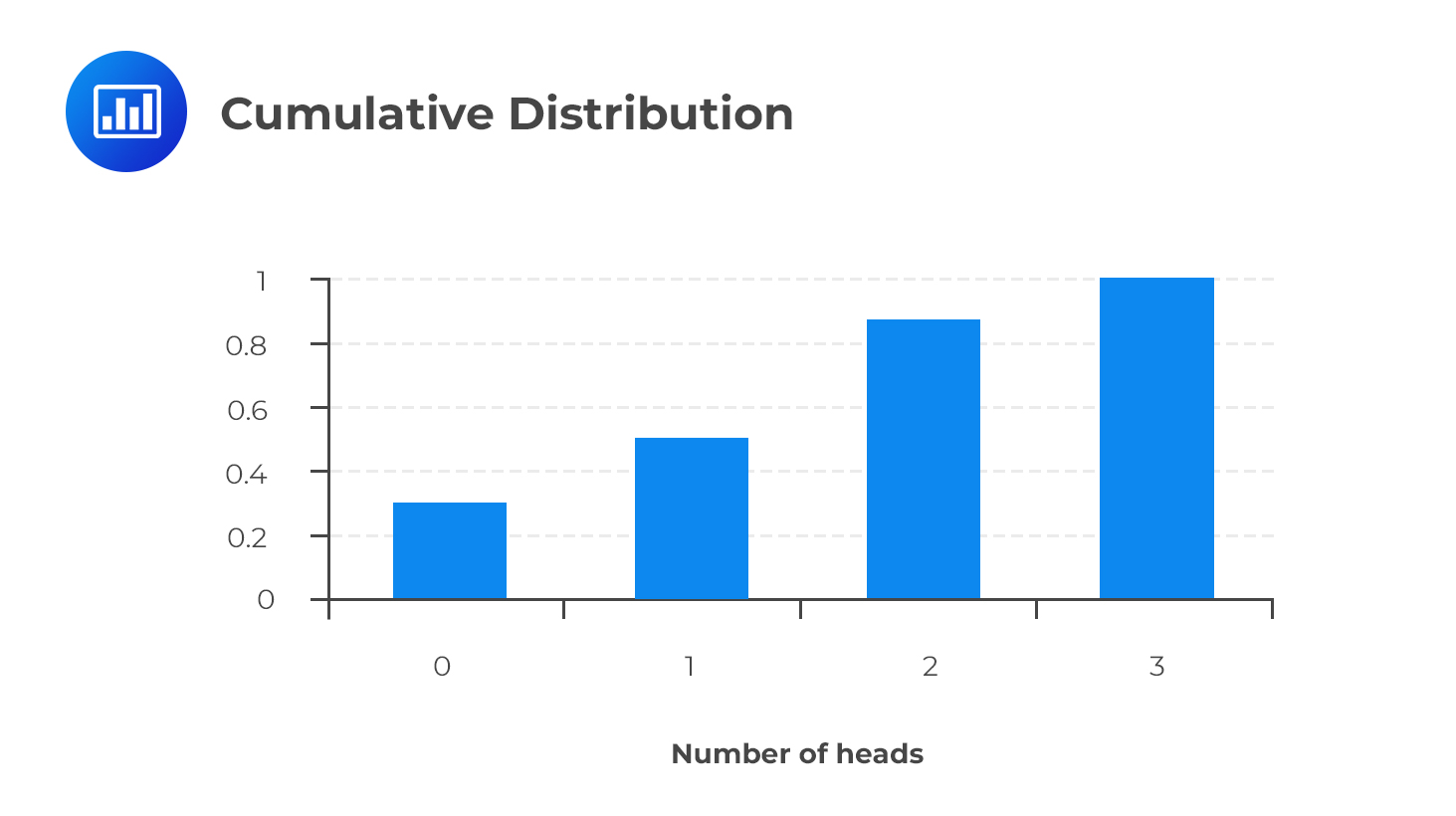# Cumulative Distribution Function (CDF)

A cumulative distribution function, F(x), gives the probability that the random variable X is less than or equal to x:

$$P(X ≤ x)$$

By analogy, this concept is very similar to the cumulative relative frequency.

A cumulative distribution is the sum of the probabilities of all values qualifying as “less than or equal to” the specified value. Perhaps an example will make this concept clearer.

### Example: Cumulative Distribution

Imagine flipping a coin three times. You would end up with the following probability distribution of the number of heads obtained:

$$\begin{array}{c|c|c|c} \text{Heads (outcomes)} & {0} & {1} & {2} & {3} \\ \hline \text{Probability} & {1/8} & {3/8} & {3/8} & {1/8} \\ \end{array}$$

To come up with a cumulative distribution function, we have to calculate the cumulative probabilities.

The cumulative probability that X is less than or equal to zero is 1/8.

To find the cumulative probability that X is less than or equal to 1, we add P(X = 0) and (P = 1):

$$P(X \le 1) =\cfrac {1}{8} + \cfrac {3}{8} = \cfrac {1}{2}$$

Similarly,

$$P(X \le 2) = \cfrac {1}{8} + \cfrac {3}{8} + \cfrac {3}{8} = \cfrac {7}{8}$$

Lastly,

$$P(X \le 3) = \cfrac {1}{8} + \cfrac {3}{8} + \cfrac {3}{8} +\cfrac {1}{8} = 1$$

$$\begin{array}{c|c|c|c} \text{Heads (outcomes)} & {0} & {1} & {2} & {3} \\ \hline \text{Probability} & {1/8} & {3/8} & {3/8} & {1/8} \\ \hline \text{Cumulative prob.} & {1/8} & {4/8} & {7/8} & {8/8} \\ \end{array}$$The CDF has two main properties:

1. all values in the CDF are between 0 and 1; and
2. the CDF either increases or remains constant as the value of the specified outcome increases.

## Interpreting the Cumulative Distribution Function

A cumulative distribution function can help us to come up with cumulative probabilities pretty easily. For example, we can use it to determine the probability of getting at least two heads, at most two heads, or even more than two heads. For example, the probability of at most two heads from the cumulative distribution above is 0.875.

## Question

Variable X can take the values 1, 2, 3, and 4. The probability of each outcome has been given below.

$$\begin{array}{c|c|c|c|c} \text{Outcome} & {1} & {2} & {3} & {4} \\ \hline \text{Probability} & {0.2} & {0.3} & {0.35} & {0.15} \\ \end{array}$$

Construct a table showing the cumulative distribution and use it to determine P(X ≤ 2).

A. 0.5

B. 0.3

C. 0.85

Solution

You simply sum up the probabilities up to and including a given outcome and come up with a table similar to the one below:

$$\begin{array}{c|c|c|c|c} \text{Heads (outcomes)} & {1} & {2} & {3} & {4} \\ \hline \text{Probability} & {0.2} & {0.3} & {0.35} & {0.15} \\ \hline \text{Cumulative prob.} & {0.2} & {0.5} & {0.85} & {1} \\ \end{array}$$

From the table, it is clear that $$P(X \le 2) = 0.5$$.

Shop CFA® Exam Prep

Offered by AnalystPrepLevel I
Level II
Level III
All Three Levels
Featured Shop FRM® Exam PrepFRM Part I
FRM Part II
FRM Part I & Part II
Learn with Us

Subscribe to our newsletter and keep up with the latest and greatest tips for success
Shop Actuarial Exams PrepExam P (Probability)
Exam FM (Financial Mathematics)
Exams P & FM
Shop GMAT® Exam PrepComplete CourseSergio Torrico
2021-07-23
Excelente para el FRM 2 Escribo esta revisión en español para los hispanohablantes, soy de Bolivia, y utilicé AnalystPrep para dudas y consultas sobre mi preparación para el FRM nivel 2 (lo tomé una sola vez y aprobé muy bien), siempre tuve un soporte claro, directo y rápido, el material sale rápido cuando hay cambios en el temario de GARP, y los ejercicios y exámenes son muy útiles para practicar.diana
2021-07-17
So helpful. I have been using the videos to prepare for the CFA Level II exam. The videos signpost the reading contents, explain the concepts and provide additional context for specific concepts. The fun light-hearted analogies are also a welcome break to some very dry content. I usually watch the videos before going into more in-depth reading and they are a good way to avoid being overwhelmed by the sheer volume of content when you look at the readings.Kriti Dhawan
2021-07-16
A great curriculum provider. James sir explains the concept so well that rather than memorising it, you tend to intuitively understand and absorb them. Thank you ! Grateful I saw this at the right time for my CFA prep.nikhil kumar
2021-06-28
Very well explained and gives a great insight about topics in a very short time. Glad to have found Professor Forjan's lectures.Marwan
2021-06-22
Great support throughout the course by the team, did not feel neglectedBenjamin anonymous
2021-05-10
I loved using AnalystPrep for FRM. QBank is huge, videos are great. Would recommend to a friendDaniel Glyn
2021-03-24
I have finished my FRM1 thanks to AnalystPrep. And now using AnalystPrep for my FRM2 preparation. Professor Forjan is brilliant. He gives such good explanations and analogies. And more than anything makes learning fun. A big thank you to Analystprep and Professor Forjan. 5 stars all the way!michael walshe
2021-03-18
Professor James' videos are excellent for understanding the underlying theories behind financial engineering / financial analysis. The AnalystPrep videos were better than any of the others that I searched through on YouTube for providing a clear explanation of some concepts, such as Portfolio theory, CAPM, and Arbitrage Pricing theory. Watching these cleared up many of the unclarities I had in my head. Highly recommended.